# Polar Coordinates and Slopes of Tangent Lines

1.   Plot the point whose polar coordinates are given.  Then find two other pairs of polar coordinates of this point, one with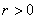and one with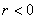.

a.)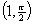b.)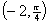c.)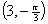2.   Plot the point whose polar coordinates are given.  Then find the Cartesian coordinates of the point.

a.)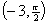b.)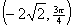c.)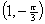3.      Given are the Cartesian coordinates of a point.  Find the polar coordinates of the point withand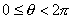.

a.)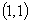b.)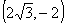c.)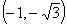4.      Sketch the region in the plane consisting of the points whose polar coordinates satisfy the given conditions.

a.)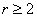b.)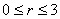,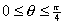c.)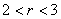,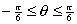5.      Find the Cartesian equation for the curve described by the given polar equation.

a.)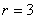b.)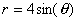6.      Find the polar equation for the curve described by the given Cartesian equation.

a.)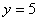b.)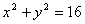7.      Sketch the curve with the given equation.

a.)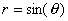b.)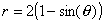c.)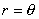d.)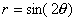e.)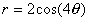8.      Find the slope of the tangent line to the given polar curve at the point specified by the value of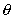.

a.)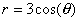,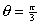b.)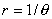,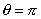c.)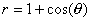,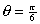9.      Find the values of, with, where the tangent line to the curve is horizontal or vertical.

a.)b.)